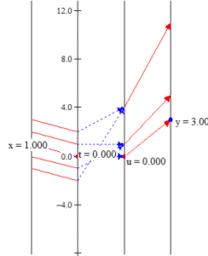# HSU Colloquium: Solve a Quadratic Equation Visually?

HSU Mathematics Department Colloquium September 13, 2018 How Many Ways Can You Solve a Quadratic Equation Visually? From the Greeks to 21st Century Technology Links: http://users.humboldt.edu/flashman/Presentations/MD.HSU.9.2018.LINKS.html Understanding a quadratic equation and finding its solutions (roots) is an important part of the curriculum where students encounter a rich domain of mathematics- including geometry, numbers (real and complex), and functions. Visualizing the solution of these equations is a valuable tool at any level. Starting with the Greek representation of the problem connected to finding areas and squares, Professor Flashman will lead a tour through various visualizations including connections to graphs and mapping diagrams and concluding with his recent work on visualizing complex functions with 3 dimensional mapping diagrams using GeoGebra.